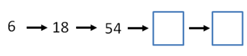Mathematics
Easy

Question

# What are the next two numbers in given sequences by following the rule?Rule: multiply 3## 162, 456    172, 516    162, 486    172, 526Hint:

## The correct answer is: 162, 486

### The given sequence is 6, 18, 54We have to find the next two terms in this sequence.Rule: Multiply by 3To find the next term, previous term is multiplied by 3We will see how it works.6 × 3 = 1818 × 3 = 54Now, let’s find the next terms.The last term from the above sequence is 54.So, we will multiply the term by 3.54 × 3 = 162This will be our new first term. We will take this term and add multiply by 3 to get the next term.162 × 3 = 486The complete sequence will be 6, 18, 54, 162, 486.And the option which is '162, 486’, is the required option.

For such questions, we should know the basic operations. When the rule used to write the pattern is not mentioned, we have to use all four operations to check the pattern. The operations are addition, subtraction, division, and multiplication.

### Related Questions to study#### With Turito Foundation.#### Get an Expert Advice From Turito.